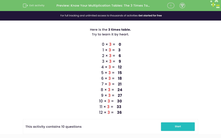# Know Your Multiplication Tables: The 3 Times Table

In this worksheet, students must recall multiplication facts from the 3 times table.Key stage:  KS 2

Curriculum topic:   Number: Multiplication and Division

Curriculum subtopic:   Use Multiplication/Division Facts (3, 4 and 8)

Popular topics:   Multiplication worksheets, Times Table worksheets

Difficulty level:#### Worksheet Overview

In this activity, we will be multiplying numbers by 3.

Here is the 3 times table.

Try to learn it by heart.

0 × 3 =   0

1 × 3 =   3

2 × 3 =   6

3 × 3 =   9

4 × 3 =   12

5 × 3 =   15

6 × 3 =   18

7 × 3 =   21

8 × 3 =   24

9 × 3 =   27

10 × 3 =   30

11 × 3 =   33

12 × 3 =   36

To multiply by 3, skip counting is a good technique.

3, 6, 9, 12, 15, 18, 21, 24, 27, 30, 33, 36

If you are stuck, choose a fact you know well to count on or back from.

So, we know that 10 × 3 = 30

Count back 3 to find 9 × 3

29, 28, 27

Count on 3 to find 11 × 3

31, 32, 33

Let's look at an example

Example

Find 4 × 3.

To find 4 lots of 3, we can skip count and choose the 4th multiple.

3, 6, 9, 12

4 × 3 = 12

Now you're ready to try some questions. Good luck!

### What is EdPlace?

We're your National Curriculum aligned online education content provider helping each child succeed in English, maths and science from year 1 to GCSE. With an EdPlace account you’ll be able to track and measure progress, helping each child achieve their best. We build confidence and attainment by personalising each child’s learning at a level that suits them.

Get started